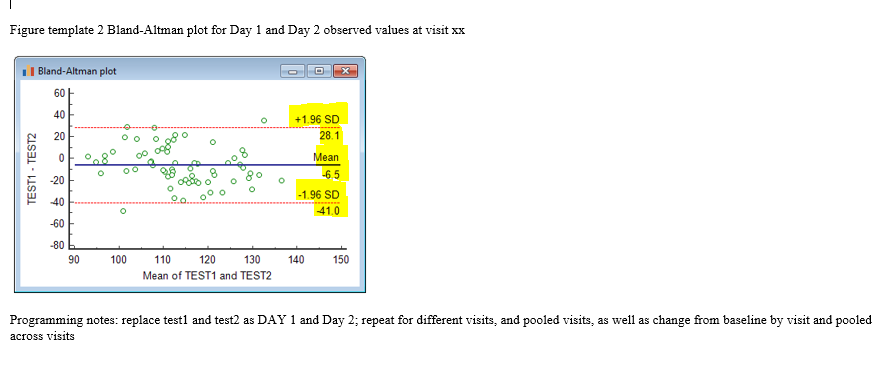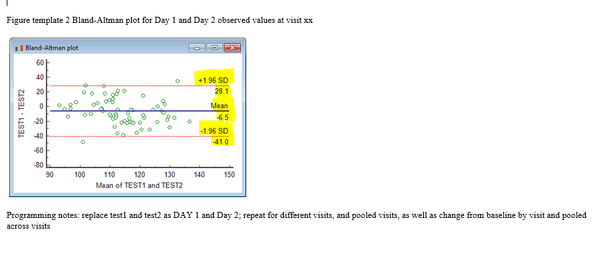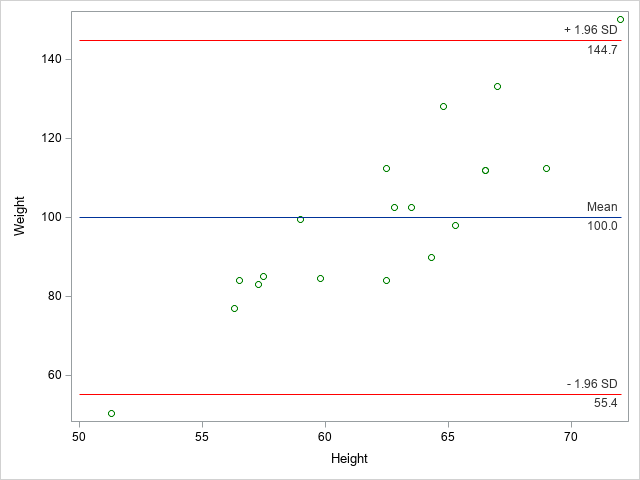## SG annotation help

Hi,

I am a novice in SAS graphics, as I don't get a lpt of practice on it.

I am trying to annotate as per the following Figure in my Figure. but somehow not able to get there. Can you please help? I will share my annotation as well, I am sure it's no good,but this is as good as I am at the moment. I have the ref lines and every other thing required here. except the annotations. Please help.

Thanks.

The expected Figure:My SAS code for Annotation:

``````/*data Anno_N;
length function \$10 x1space \$12 label TEXTFONT \$500;
set datafile2 end=last;
*retain id 0 function x1space y1space textcolor textsize TEXTFONT
textweight anchor width widthunit y1;
TEXTFONT="Albany AMT";
if newcol=mdiffm1m2 then do;
textcolor="black"; function='text'; y1=mdiffm1m2; x1space="Wallpercent"; y1space="datavalue";
label="Mean"; widthunit='percent'; textsize=10; textweight='normal'; anchor='right';
end;
if newcol=lldiffm1m22 then do;
textcolor="black"; function='text'; y2=lldiffm1m22; x2space="Wallpercent"; y2space="datavalue";
label="Mean3"; widthunit='percent'; textsize=10; textweight='normal'; anchor='right';width=30;
end;
run;*/

options nonumber nodate;
ods graphics / reset width=1200px height=700px imagename='Full' ;
ods listing close;
ods escapechar='~';
ODS rtf FILE = "&outpath.&output..rtf";
symbol value=dot interpol=join repeat=100;
ods graphics / reset width=1200px height=700px imagename='Full';

title1 j=c  "Bland-Altman Plot for Day 1 and Day 2 observed values at Screening, 6 Min WT Dist.";
title2 j=c "Efficacy Set";
footnote1 " ";

proc sgplot data=datafile2(where=(_visit_="Screening")) /*sganno=anno_n*/;
scatter x=meanm1m2 y=diffm1m2/ markerattrs=(color=green) tip=(meanm1m2 diffm1m2);
refline lldiffm1m22 / axis=y lineattrs=(color=red pattern=4 thickness=2pt);
refline mdiffm1m2/ axis=y lineattrs=(color=blue thickness=2pt);
refline uldiffm1m22/ axis=y lineattrs=(color=red pattern=4 thickness=2pt);
yaxis label='Day 1 - Day 2' labelattrs=(style=normal );
xaxis label='Mean of Day 1 and Day 2';
run;

ods rtf close;
ods listing;``````

The SG plot seems to be okay. I will uncomment sganno if my annotation works.  but this doesn't.

Let me know, if ant other info is needed. Thanks

1 ACCEPTED SOLUTION

Accepted Solutions

## Re: SG annotation help

Consider skipping it as an annotation and use a TEXT statement instead.

For text, create three new variables, your text, x and y locations.

Then use a TEXT statement within the SGPLOT procedure.

https://documentation.sas.com/?docsetId=grstatproc&docsetTarget=n0lprj1bdrlrkgn1vmqnd7r6fnry.htm&doc...

``````data datafile3;
set datafile2 anno_n;

run;

[rest of sas code]

proc sgplot data=datafile3(where=(_visit_ = "Screening"));

[rest of SAS code]

text text = label x= Xvariable y = YVariable ;

run;``````

@VinnyR wrote:

Hi,

I am a novice in SAS graphics, as I don't get a lpt of practice on it.

I am trying to annotate as per the following Figure in my Figure. but somehow not able to get there. Can you please help? I will share my annotation as well, I am sure it's no good,but this is as good as I am at the moment. I have the ref lines and every other thing required here. except the annotations. Please help.

Thanks.

The expected Figure:My SAS code for Annotation:

``````/*data Anno_N;
length function \$10 x1space \$12 label TEXTFONT \$500;
set datafile2 end=last;
*retain id 0 function x1space y1space textcolor textsize TEXTFONT
textweight anchor width widthunit y1;
TEXTFONT="Albany AMT";
if newcol=mdiffm1m2 then do;
textcolor="black"; function='text'; y1=mdiffm1m2; x1space="Wallpercent"; y1space="datavalue";
label="Mean"; widthunit='percent'; textsize=10; textweight='normal'; anchor='right';
end;
if newcol=lldiffm1m22 then do;
textcolor="black"; function='text'; y2=lldiffm1m22; x2space="Wallpercent"; y2space="datavalue";
label="Mean3"; widthunit='percent'; textsize=10; textweight='normal'; anchor='right';width=30;
end;
run;*/

options nonumber nodate;
ods graphics / reset width=1200px height=700px imagename='Full' ;
ods listing close;
ods escapechar='~';
ODS rtf FILE = "&outpath.&output..rtf";
symbol value=dot interpol=join repeat=100;
ods graphics / reset width=1200px height=700px imagename='Full';

title1 j=c  "Bland-Altman Plot for Day 1 and Day 2 observed values at Screening, 6 Min WT Dist.";
title2 j=c "Efficacy Set";
footnote1 " ";

proc sgplot data=datafile2(where=(_visit_="Screening")) /*sganno=anno_n*/;
scatter x=meanm1m2 y=diffm1m2/ markerattrs=(color=green) tip=(meanm1m2 diffm1m2);
refline lldiffm1m22 / axis=y lineattrs=(color=red pattern=4 thickness=2pt);
refline mdiffm1m2/ axis=y lineattrs=(color=blue thickness=2pt);
refline uldiffm1m22/ axis=y lineattrs=(color=red pattern=4 thickness=2pt);
yaxis label='Day 1 - Day 2' labelattrs=(style=normal );
xaxis label='Mean of Day 1 and Day 2';
run;

ods rtf close;
ods listing;``````

The SG plot seems to be okay. I will uncomment sganno if my annotation works.  but this doesn't.

Let me know, if ant other info is needed. Thanks

13 REPLIES 13

## Re: SG annotation help

Consider skipping it as an annotation and use a TEXT statement instead.

For text, create three new variables, your text, x and y locations.

Then use a TEXT statement within the SGPLOT procedure.

https://documentation.sas.com/?docsetId=grstatproc&docsetTarget=n0lprj1bdrlrkgn1vmqnd7r6fnry.htm&doc...

``````data datafile3;
set datafile2 anno_n;

run;

[rest of sas code]

proc sgplot data=datafile3(where=(_visit_ = "Screening"));

[rest of SAS code]

text text = label x= Xvariable y = YVariable ;

run;``````

@VinnyR wrote:

Hi,

I am a novice in SAS graphics, as I don't get a lpt of practice on it.

I am trying to annotate as per the following Figure in my Figure. but somehow not able to get there. Can you please help? I will share my annotation as well, I am sure it's no good,but this is as good as I am at the moment. I have the ref lines and every other thing required here. except the annotations. Please help.

Thanks.

The expected Figure:My SAS code for Annotation:

``````/*data Anno_N;
length function \$10 x1space \$12 label TEXTFONT \$500;
set datafile2 end=last;
*retain id 0 function x1space y1space textcolor textsize TEXTFONT
textweight anchor width widthunit y1;
TEXTFONT="Albany AMT";
if newcol=mdiffm1m2 then do;
textcolor="black"; function='text'; y1=mdiffm1m2; x1space="Wallpercent"; y1space="datavalue";
label="Mean"; widthunit='percent'; textsize=10; textweight='normal'; anchor='right';
end;
if newcol=lldiffm1m22 then do;
textcolor="black"; function='text'; y2=lldiffm1m22; x2space="Wallpercent"; y2space="datavalue";
label="Mean3"; widthunit='percent'; textsize=10; textweight='normal'; anchor='right';width=30;
end;
run;*/

options nonumber nodate;
ods graphics / reset width=1200px height=700px imagename='Full' ;
ods listing close;
ods escapechar='~';
ODS rtf FILE = "&outpath.&output..rtf";
symbol value=dot interpol=join repeat=100;
ods graphics / reset width=1200px height=700px imagename='Full';

title1 j=c  "Bland-Altman Plot for Day 1 and Day 2 observed values at Screening, 6 Min WT Dist.";
title2 j=c "Efficacy Set";
footnote1 " ";

proc sgplot data=datafile2(where=(_visit_="Screening")) /*sganno=anno_n*/;
scatter x=meanm1m2 y=diffm1m2/ markerattrs=(color=green) tip=(meanm1m2 diffm1m2);
refline lldiffm1m22 / axis=y lineattrs=(color=red pattern=4 thickness=2pt);
refline mdiffm1m2/ axis=y lineattrs=(color=blue thickness=2pt);
refline uldiffm1m22/ axis=y lineattrs=(color=red pattern=4 thickness=2pt);
yaxis label='Day 1 - Day 2' labelattrs=(style=normal );
xaxis label='Mean of Day 1 and Day 2';
run;

ods rtf close;
ods listing;``````

The SG plot seems to be okay. I will uncomment sganno if my annotation works.  but this doesn't.

Let me know, if ant other info is needed. Thanks

## Re: SG annotation help

``````proc sort data=datafile3;
by rstestcd meanm1m2;
run;

data anno11(keep=rstestcd col1 ) anno22(keep=rstestcd col2 ) anno33(keep=rstestcd _visit_ usubjid newcol_ y1 y2 texty1 texty2);
set datafile3;
by rstestcd meanm1m2;
length texty1 texty2 \$ 20;;
if newcol_=mdiffm1m2_ then do; y1=mdiffm1m2_+0.5; y2=mdiffm1m2_-0.5; texty1="Mean"; texty2=strip(put(mdiffm1m2_,best.)); end;
if newcol_=lldiffm1m22_ then do; y1=lldiffm1m22_+0.5; y2=lldiffm1m22_-0.5; texty1="-1.96 SD"; texty2=strip(put(lldiffm1m22_,best.)); end;
if newcol_=uldiffm1m22_ then do; y1=uldiffm1m22_+0.5; y2=uldiffm1m22_-0.5; texty1="+1.96 SD"; texty2=strip(put(uldiffm1m22_,best.)); end;
output anno33;
if last.rstestcd then do; col1=meanm1m2;output anno11;end;
if first.rstestcd then do;  col2=meanm1m2;output anno22;end;
run;

data anno_1;
merge anno11 anno22;
by rstestcd;
x=((col1-col2)*4.9/5)+col2;
run;

data f31;
merge datafile3 anno_1;
by rstestcd;
run;

proc sort data=anno33;
by _visit_ rstestcd usubjid newcol_;
run;

proc sort data=f31;
by _visit_ rstestcd usubjid newcol_;
run;

data f32;
merge f31 anno33;
by _visit_ rstestcd usubjid newcol_;
run;

options nonumber nodate;
ods graphics / reset width=1200px height=700px imagename='Full' ;
ods listing close;
ods escapechar='~';
ODS rtf FILE = "&outpath.&output..rtf";
symbol value=dot interpol=join repeat=100;
ods graphics / reset width=1200px height=700px imagename='Full';

title1 j=c  "Bland-Altman Plot for Day 1 and Day 2 observed values at Screening, NSAA Total Score";
title2 j=c "Efficacy Set";
footnote1 " ";

proc sgplot data=f2(where=(_visit_="Screening")) noautolegend /*sganno=anno_n*/;
scatter x=meanm1m2 y=diffm1m2/ markerattrs=(color=green) tip=(meanm1m2 diffm1m2);
refline lldiffm1m22 / axis=y lineattrs=(color=red pattern=4 thickness=2pt);
refline mdiffm1m2/ axis=y lineattrs=(color=blue thickness=2pt);
refline uldiffm1m22/ axis=y lineattrs=(color=red pattern=4 thickness=2pt);
yaxis label='Day 1 - Day 2' labelattrs=(style=normal );
xaxis label='Mean of Day 1 and Day 2';
text x=x y=y1 text=texty1/ textattrs=(color=gray size=6pt);
text x=x y=y2 text=texty2/ textattrs=(color=gray size=6pt);
run;

ods rtf close;
ods listing;``````

## Re: SG annotation help

Does this mean you have it working or you need further assistance? You have code - we can't run it without the same data. If you're having issues remember to include the log as well.

## Re: SG annotation help

Unless having the two bits of text above and below the reference line is super critical then perhaps just using a LABEL with the proper options such as:

```proc sgplot data=sashelp.class;
scatter x=height y=weight;
refline 95 / axis=Y label="Meaning text*Ref @ 95"
labelloc=inside  labelpos=max
splitchar='*' splitjustify= right
;
run;```

The splitchar  will vertically split the text of the label at the *, the justification set both pieces to display right justified and labelloc and labelpos put the text at the right side of the reference line inside the graph. Labelattrs options would let you set the text color, font family, size, style and weight. Or use a reference to a style attribute such as GraphTitleText .

## Re: SG annotation help

I modified my code like this:

proc sgplot data=datafile2(where=(_visit_="Screening")) /*sganno=anno_n*/;
scatter x=meanm1m2 y=diffm1m2/ markerattrs=(color=green) tip=(meanm1m2 diffm1m2);
refline lldiffm1m22 / axis=y lineattrs=(color=red pattern=4 thickness=2pt)
label="Mean * lldiffm1m22" labelloc=inside labelpos=max splitchar="*" splitjustify=right;
refline mdiffm1m2/ axis=y lineattrs=(color=blue thickness=2pt);
refline uldiffm1m22/ axis=y lineattrs=(color=red pattern=4 thickness=2pt);
yaxis label='Day 1 - Day 2' labelattrs=(style=normal );
xaxis label='Mean of Day 1 and Day 2';
run;

It didn't work...I tried ti use labek yesterday as well, but it didn't work...I don't know why.

## Re: SG annotation help

Is there an error in the log?

No.....no error

## Re: SG annotation help

@VinnyR wrote:

No.....no errorDanH_sas
SAS Super FREQ

## Re: SG annotation help

Your annotate code has inputs from another data set. Make sure your rename any variables you use from that data set to names used by SGANNO, or your annotation will not work. I created a simple example below that simulates what you're trying to accomplish.

Hope this helps!
Dan

``````data anno;
length anchor \$ 11;
retain id "myid" function "text" x1space "wallpercent" y1space "datavalue" x1 100 width 40;
input label \$ 1-16 y1 anchor \$;
cards;
The top label     65 bottomright
The bottom label  65 topright
;
run;

proc sgplot data=sashelp.class sganno=anno;
scatter x=weight y=height;
refline 65;
run;``````

## Re: SG annotation help

@VinnyR wrote:

I modified my code like this:

proc sgplot data=datafile2(where=(_visit_="Screening")) /*sganno=anno_n*/;
scatter x=meanm1m2 y=diffm1m2/ markerattrs=(color=green) tip=(meanm1m2 diffm1m2);
refline lldiffm1m22 / axis=y lineattrs=(color=red pattern=4 thickness=2pt)
label="Mean * lldiffm1m22" labelloc=inside labelpos=max splitchar="*" splitjustify=right;
refline mdiffm1m2/ axis=y lineattrs=(color=blue thickness=2pt);
refline uldiffm1m22/ axis=y lineattrs=(color=red pattern=4 thickness=2pt);
yaxis label='Day 1 - Day 2' labelattrs=(style=normal );
xaxis label='Mean of Day 1 and Day 2';
run;

It didn't work...I tried ti use labek yesterday as well, but it didn't work...I don't know why.

Doesn't work is awful vague.

Are there errors in the log?: Post the code and log in a code box opened with the {i} to maintain formatting of error messages.

Unexpected output? Provide input data in the form of data step code pasted into a code box, the actual results and the expected results. Instructions here: https://communities.sas.com/t5/SAS-Communities-Library/How-to-create-a-data-step-version-of-your-dat... will show how to turn an existing SAS data set into data step code that can be pasted into a forum code box using the {i} icon or attached as text to show exactly what you have and that we can test code against.

Could you run the exact code that I showed using the SASHELP.CLASS data set? If that runs and your code doesn't then you may have an issue with your data.

If you mean that the LABEL text did not have the value of a variable then you need to modify the value of the variable. If you use Label="some text* variablename" then the text inside the quotes is what you get: "some text variablename" though the variable name should be on a second line. Text or Variable, not both.

You may need to use a macro variable to have the value in the Label text as the SPLITCHAR option does not appear to apply to variable values, only literal text. Which may make @Reeza's suggestion more attractive.

## Re: SG annotation help

```1624
1625
1626
1627  options nonumber nodate;
1628  ods graphics / reset width=1200px height=700px
1628! imagename='Full' ;
1629  ods listing close;
1630  ods escapechar='~';
1631  ODS rtf FILE = "&outpath.&output..rtf";
NOTE: Writing RTF Body file: S:\statpgm\dev\avi-4658\4658-301\final\outputs\adhoc\sample.rtf
1632  symbol value=dot interpol=join repeat=100;
1633  ods graphics / reset width=1200px height=700px imagename='Full';
1634
1635  title1 j=c  "Bland-Altman Plot for Day 1 and Day 2 observed values at Screening, 6 Min WT
1635!  Dist.";
1636  title2 j=c "Efficacy Set";
1637  footnote1 " ";
1638
1639  proc sgplot data=datafile2(where=(_visit_="Screening")) /*sganno=anno_n*/;
1640  scatter x=meanm1m2 y=diffm1m2/ markerattrs=(color=green) tip=(meanm1m2 diffm1m2);
1641  refline lldiffm1m22 / axis=y lineattrs=(color=red pattern=4 thickness=2pt)
1642        label="Mean * lldiffm1m22" labelloc=inside labelpos=max splitchar="*"
1642! splitjustify=right;
1643  refline mdiffm1m2/ axis=y lineattrs=(color=blue thickness=2pt);
1644  refline uldiffm1m22/ axis=y lineattrs=(color=red pattern=4 thickness=2pt);
1645  yaxis label='Day 1 - Day 2' labelattrs=(style=normal );
1646  xaxis label='Mean of Day 1 and Day 2';
1647  run;

NOTE: PROCEDURE SGPLOT used (Total process time):
real time           1.49 seconds
cpu time            0.59 seconds

WARNING: WIDTH exceeds available space for RTF destination. Setting WIDTH=11520px.
NOTE: CURVELABEL='Mean * lldiffm1m22' is invalid. The option value cannot be a string when a
column of reference lines is plotted. The label will not be drawn.
NOTE: CURVELABEL='Mean * lldiffm1m22' is invalid. The option value cannot be a string when a
column of reference lines is plotted. The label will not be drawn.
NOTE: There were 327 observations read from the data set
WORK.DATAFILE2.
WHERE _visit_='Screening';

1648
1649  ods rtf close;
1650  ods listing;```## Re: SG annotation help

The label probably works only if refline variable is a constant(number). Try using refline with variable name, then label does not work. Also, I did not understand the note from the log I posted.

I really appreciate everyone's help. I am trying the other methods posted as well.

Thanks,

Vinny

## Re: SG annotation help

Here's a complete example, using proc sql to calculate the statistics, and using annotate to draw the lines and the labels:

proc sql noprint;
create table my_data as
select unique *, avg(weight) as mean, std(weight) as sd
from sashelp.class;
create table my_anno as select unique mean, sd
from my_data;
quit; run;

data my_anno; set my_anno;
layer='front';

/* annotate the lines */
x1space='datapercent'; y1space='datavalue';
x2space='datapercent'; y2space='datavalue';
function='line'; linethickness=1;
linecolor='red'; x1=0; y1=mean+1.96*sd; x2=100; y2=mean+1.96*sd; output;
linecolor='blue'; x1=0; y1=mean; x2=100; y2=mean; output;
linecolor='red'; x1=0; y1=mean-1.96*sd; x2=100; y2=mean-1.96*sd; output;
/* annotate the text labels above & below each line */
function='text'; textcolor='gray33'; textsize=9; textweight='normal';
width=100; widthunit='percent';
x1=100; y1=mean+1.96*sd;
anchor='bottomright'; label='+ 1.96 SD'; output;
anchor='topright'; label=trim(left(put(mean+1.96*sd,comma5.1))); output;
x1=100; y1=mean;
anchor='bottomright'; label='Mean'; output;
anchor='topright'; label=trim(left(put(mean,comma5.1))); output;
x1=100; y1=mean-1.96*sd;
anchor='bottomright'; label='- 1.96 SD'; output;
anchor='topright'; label=trim(left(put(mean-1.96*sd,comma5.1))); output;
run;

proc sgplot data=my_data sganno=my_anno;
scatter y=weight x=height / markerattrs=(color=green) tip=(weight height);
run;Discussion stats
• 13 replies
• 2035 views
• 7 likes
• 5 in conversation